# Image Denoising and Segmentation Using Markov Random Field

Date:

In this project, we Optimized an Energy Function using Simulated Annealing to predict real value of each pixel. We used different Color Spaces in this project. This project has two parts. In part one, we use markov random field to denoise an image. In Part two, we use similar model for image segmentation.

For checking the code, click on Codes

Markov Random Field Models provide a simple and effective way to model the spatial dependencies in image pixels.
So we useed them to model the connection between two neighbour pixels.
In our problem we have to define an energy function on hidden states corresponding to true values of each pixels, then we minimize this function to obtain the best prediction.
Our energy function is defined as below: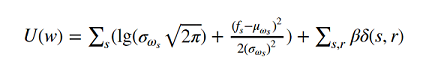## Denoising

a_complete_set_for_part_1(arr, max_iter=1e7, var=1e4, betha=1e4)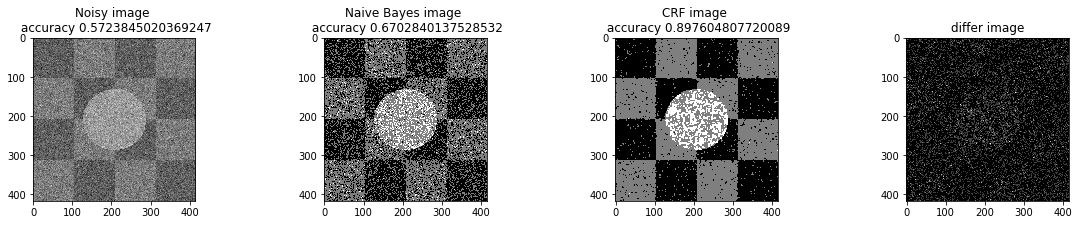a_complete_set_for_part_1_some_pixels_known(arr,  known_index, max_iter=1e6, var=1e4, betha=bta)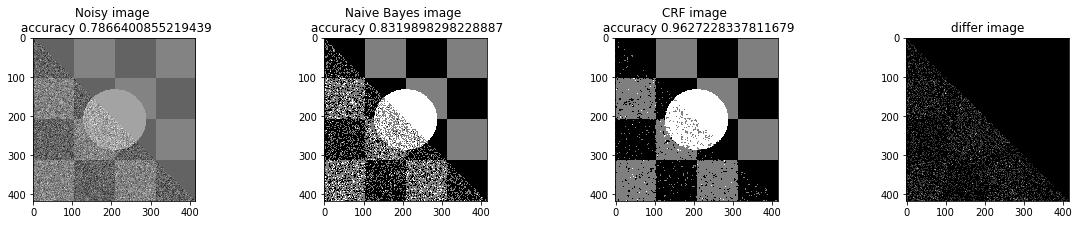## Image Segmentation

In this part, we used Markov Random Field for image segmentation.
We used different image color space:

• Gray Scale
• HSV
• RGB Format

### Gray Scale

a_complete_set_for_part_2(arr,class_info, max_iter=1e7, betha=1e6)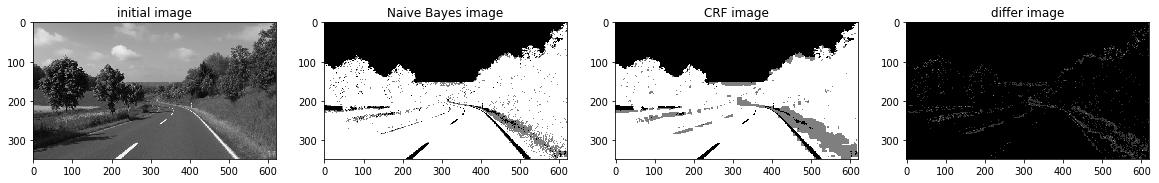### HSV color space

Now we want to use HSV color space for training our data.

a_complete_set_for_part_2(arr_h,class_info, max_iter=1e6, betha=1e6)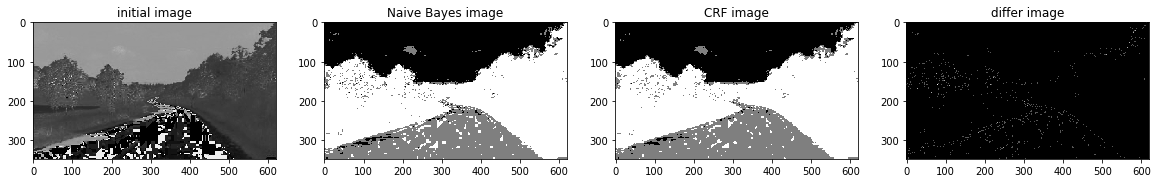### RGB color space

In this part, we used RGB color format in training since there is some information that can be captured by pixels colors.
We used RGB values in potential function.

a_complete_set_for_part_2_3_color(max_iter=1e6, betha=1e6)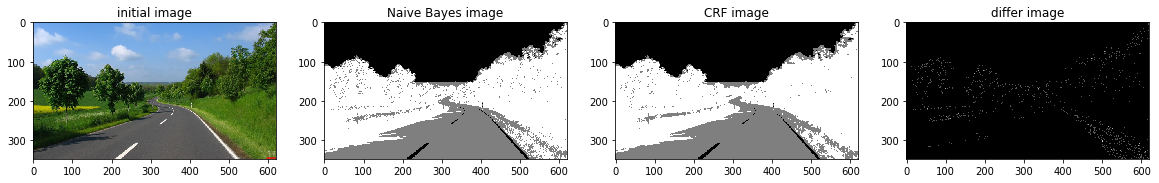a_complete_set_for_part_2_3_color(max_iter=1e6, betha=1e6,
schedule=linear_multiplicative_cooling_schedule, temprature_function_constant=0.5)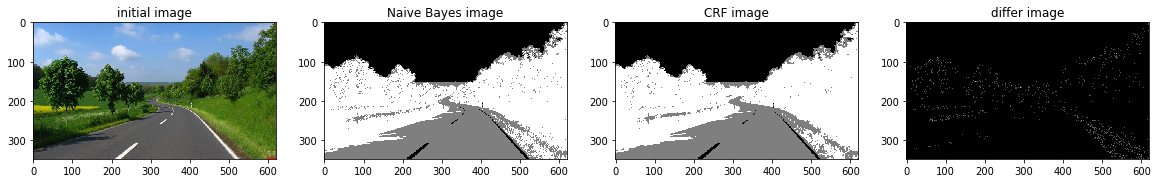## Conclusion

Grayscale image format didn’t have sufficient information for CRF models in this task.
The value H in HSV image format had better information for segmentation using CRF models. And the result was better.
The RGB format also had good information for segmenting the image. Because these three segments have different colors. So if a CRF model considers colors of the image for classification, then the result is going to be better compared to Grayscale images.